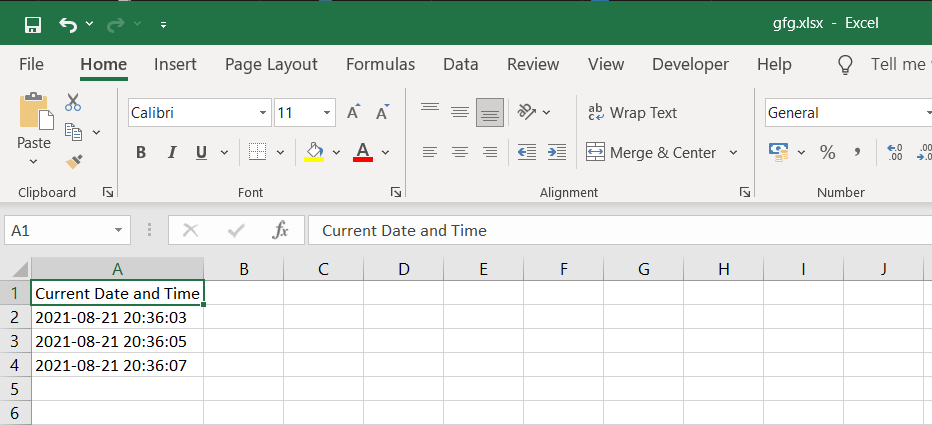# How to add timestamp to excel file in Python

In this article, we will discuss how to add a timestamp to an excel file using Python.

## Modules required

• datetime: This module helps us to work with dates and times in Python.
`pip install datetime`
• openpyxl: It is a Python library used for reading and writing Excel files.
`pip install openpyxl`
• time: This module provides various time-related functions.

## Stepwise Implementation

Step 1: Create the workbook object and select the active sheet:

```wb = Workbook()
ws = wb.active```

Step 2 (Optional): Write the heading in cell A1.

```# Here column=1 represents column A and row=1 represents first row.
ws.cell(row=1, column=1).value = "Current Date and Time"```

Step 3: Use the following command to get the current DateTime from the system.

`time = datetime.datetime.now().strftime('%Y-%m-%d %H:%M:%S')`

The strftime() function is used to convert date and time objects to their string representation. It takes one or more inputs of formatted code and returns the string representation.

Step 4: Write the DateTime in cell A2.

`ws.cell(row=2, column=1).value = time`

Step 5: Initiate a sleep of 2 seconds using time.sleep(2)

Step 6: Similarly, get the current datetime again and write it in cell A3. Here you will notice a difference of 2 seconds from the previous DateTime since there was a sleep of 2 seconds in between.

Step 7: Finally, Save the excel workbook with a file name and close the workbook

```wb.save('gfg.xlsx')
wb.close()```

Below is the full implementation:

## Python3

 `# Import the required modules ` `import` `datetime ` `from` `openpyxl ``import` `Workbook ` `import` `time ` ` `  ` `  `# Main Function ` `if` `__name__ ``=``=` `'__main__'``: ` `   `  `    ``# Create a workbook object ` `    ``wb ``=` `Workbook() ` ` `  `    ``# Select the active sheet ` `    ``ws ``=` `wb.active ` ` `  `    ``# Heading of Cell A1 ` `    ``ws.cell(row``=``1``, column``=``1``).value ``=` `"Current Date and Time"` ` `  `    ``# Cell A2 containing the Current Date and Time ` `    ``ws.cell(row``=``2``, column``=``1``).value ``=` `datetime.datetime.now().strftime(``'%Y-%m-%d %H:%M:%S'``) ` ` `  `    ``# Sleep of 2 seconds ` `    ``time.sleep(``2``) ` ` `  `    ``# Cell A3 containing the Current Date and Time ` `    ``ws.cell(row``=``3``, column``=``1``).value ``=` `datetime.datetime.now().strftime(``'%Y-%m-%d %H:%M:%S'``) ` `    ``time.sleep(``2``) ` ` `  `    ``# Cell A4 containing the Current Date and Time ` `    ``ws.cell(row``=``4``, column``=``1``).value ``=` `datetime.datetime.now().strftime(``'%Y-%m-%d %H:%M:%S'``) ` ` `  `    ``# Save the workbook with a ` `    ``# filename and close the object ` `    ``wb.save(``'gfg.xlsx'``) ` `    ``wb.close()`

Output:Whether you're preparing for your first job interview or aiming to upskill in this ever-evolving tech landscape, GeeksforGeeks Courses are your key to success. We provide top-quality content at affordable prices, all geared towards accelerating your growth in a time-bound manner. Join the millions we've already empowered, and we're here to do the same for you. Don't miss out - check it out now!

Previous
Next